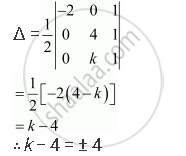Share

# Find Values Of K If Area of Triangle is 4 Square Units and Vertices Are (−2, 0), (0, 4), (0, K) - CBSE (Commerce) Class 12 - Mathematics

#### Question

Find values of k if area of triangle is 4 square units and vertices are (−2, 0), (0, 4), (0, k)

#### Solution

The area of the triangle with vertices (−2, 0), (0, 4), (0, k) is given by the relation,When k − 4 = − 4, k = 0.

When k − 4 = 4, k = 8.

Hence, k = 0, 8.

Is there an error in this question or solution?

#### Video TutorialsVIEW ALL 

Solution Find Values Of K If Area of Triangle is 4 Square Units and Vertices Are (−2, 0), (0, 4), (0, K) Concept: Area of a Triangle.
S